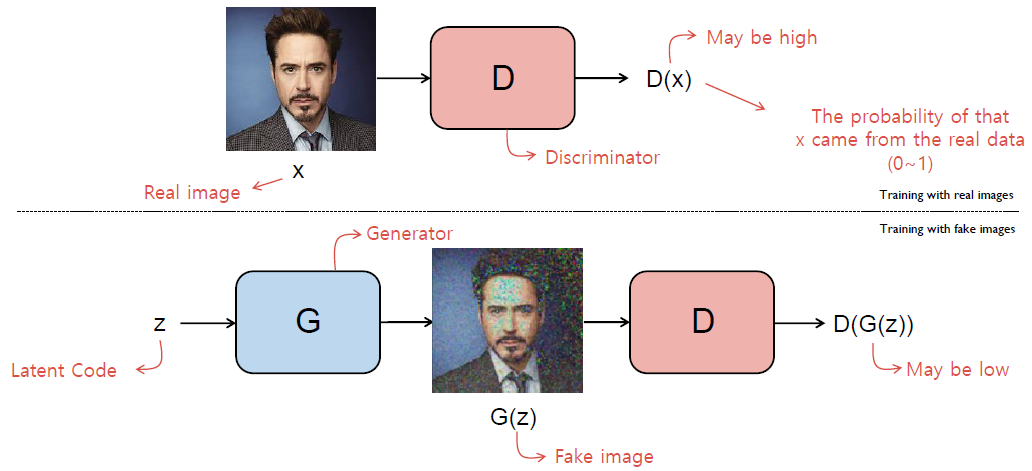### Recent Posts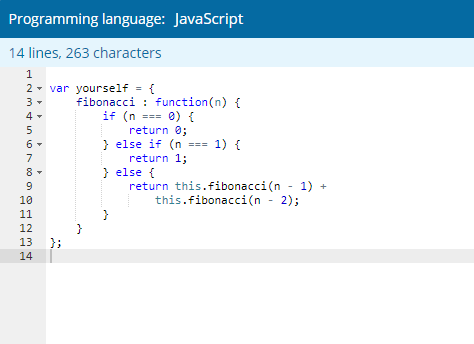## Codility Lesson14: MinMaxDivision

Sharing an answer code of mine about MinMaxDivision problem of Codility lesson 14.## Codility Lesson17: NumberSolitaire

Sharing an answer code of mine about NumberSolitaire problem of Codility lesson 17.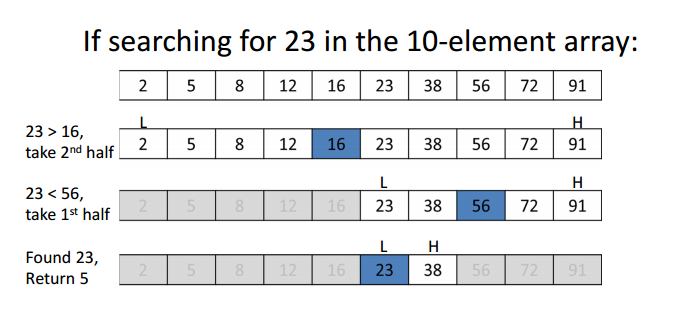## Binary Search

Learn about Binary Search which is a simple and very useful algorithm whereby many linear algorithms can be optimized to run in logarithmic time.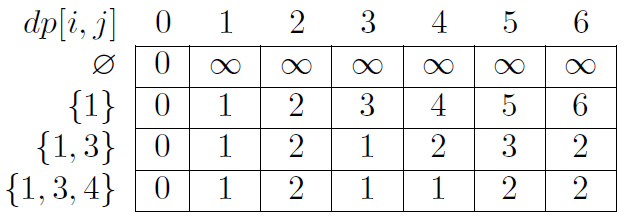## Dynamic Programming

Learn about Dynamic Programming which is a famous and important algorithm for solving problems.## Codility Lesson16: TieRopes

Sharing an answer code of mine about TieRopes problem of Codility lesson 16.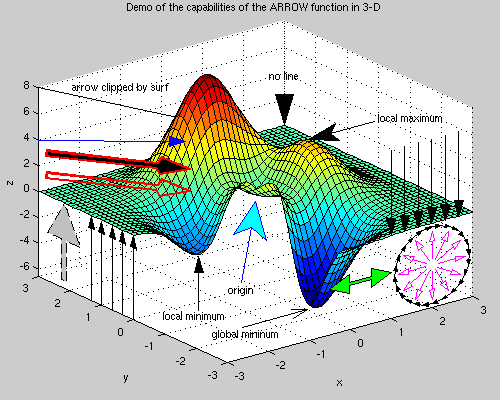## AI Related Terms

This post will be about artificial intelligence related terms including linear algebra, probability distribution, machine learning and deep learning## Codility Lesson15: MinAbsSumOfTwo